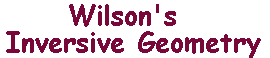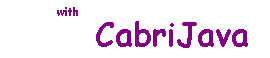Inversion in a circle
 Our version of Apollonius's Theorem led us to make the Definition Suppose that C is a circle with centre O and radius r, and that P is any point other than O. Then the inverse of P with repsect to C is the point P' such that (1) P' lies on the ray OP, and (2) OP.OP' = r2. We denote the inverse by iC(P), or, if C is obvious, by P'. Inversion is built into Cabri. This CabriJava window allows you to experiment with inversion in the blue circle. As you drag the point P, watch what happens to the inverse point P'. You can also vary the circle by dragging the centre or a point on the perimeter. Basic properties P = P' if and only if P lies on C. P is inside C if and only if P' is outside C. iC has order 2 (i.e. (P')' = P. These follow easily from the definition. There are, however, two problems: iC(O) is undefined, and there is no P with iC(P)=O. These occur since we cannot have OO.OP = r2. To motivate the way we resolve this, you can use the CabriJava pane to investigate what happens as P approaches O. You should conclude that P' moves rapidly out along the ray OP.In an intuitive sense, P' approaches infinity. In fact, when P reaches O, The ray (and hence P') are undefined. See The extended plane